# What Is The Riemann Hypothesis? And Why Do People Want To Solve It?Published3Comments270Shares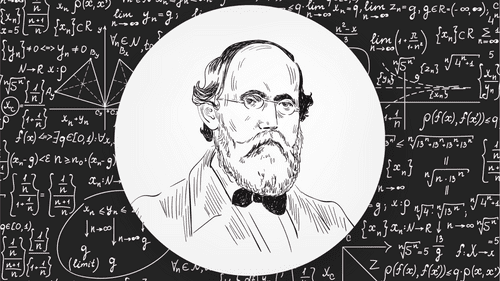Bernhard Riemann found an interesting property of his function and moved on. 162 years later, it's one of the most important unsolved problems in mathematics. Image credit: Natata/Shutterstock.com Marina Sun/Shutterstock.com

"Ask any professional mathematician what is the single most important open problem in the entire field," wrote mathematician Keith Devlin in 1998, "and you are virtually certain to receive the answer 'the Riemann Hypothesis'".

The Riemann hypothesis has been the “holy grail of mathematics” since it was first conjectured in 1859. It was one of David Hilbert’s 23 problems in 1900 and one of the seven Millennium Prize problems a century later.

It’s been called “the most famous unsolved problem … in all of mathematics”, and for good reason: it has dozens of books devoted to it, shows up on TV, and has a semi-regular slot in the news cycle.

But what is it? Why do people keep trying to prove it? And what happens if they do?

Time to take a deep dive into math and see if we can make some sense of the Riemann hypothesis.

Is the Riemann hypothesis hard to understand?

There often seems to be an unwritten rule that the harder a math problem is, the easier it looks to a layperson. Fermat’s Last Theorem, for example, took more than 350 years to prove, and it can be expressed in a single sentence.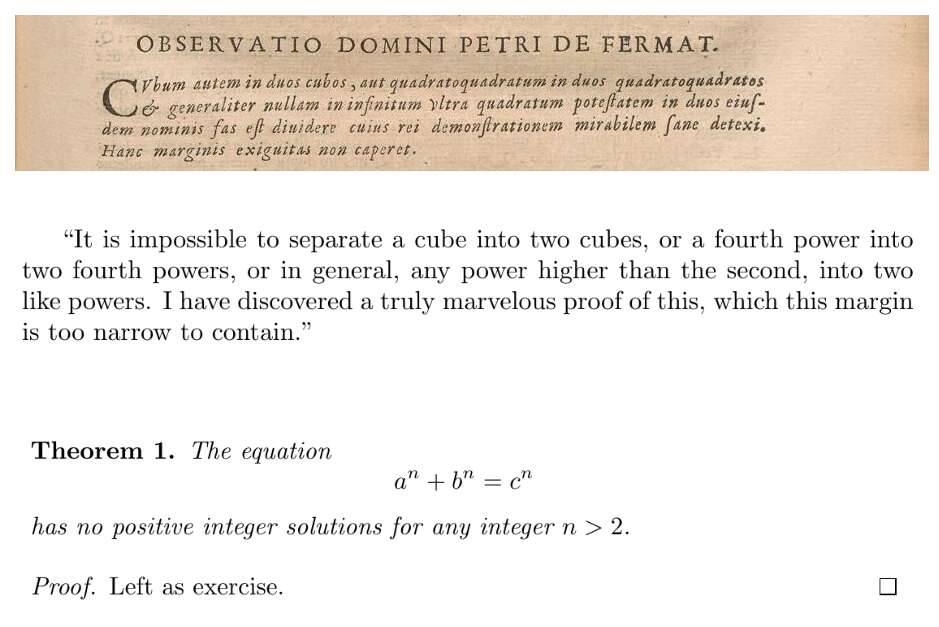Fermat's Last Theorem: as he wrote it, translated into English, and written in mathematical formulation.

The Riemann hypothesis is a notable exception. To even understand the statement of the conjecture, you need at least some knowledge of complex analysis and analytic number theory – not to mention the ability to read mathematical shorthand, which can often be a language unto itself.

But this wouldn’t be much of an explainer if we left it at that – so let’s go for a crash course in prime number theory and figure out some idea of what this 160-year-old puzzle actually means.

Why are prime numbers involved?

Before you can understand why the Riemann hypothesis matters, you have to understand what prime numbers are. You might remember your elementary school math teacher describing them as numbers that can only be divided by themselves and one, and that’s true, but that’s not all they are. To professional mathematicians, this property makes them incredibly important: they’re basically the atoms of math. Just as (theoretically, at least) any physical item can be split into its constituent atoms, any integer you can think of can be split into a unique set of prime factors. To pick a random example, 231 can be expressed as the product of 3, 7, and 11.

That’s important, and not just because it makes mathematicians feel all warm and fuzzy inside. This kind of math is used to send encrypted messages over the internet: it’s called RSA encryption, and it works based on the idea that it’s much harder to break a large number into its prime factors than it is to take a bunch of prime factors and find what large number they multiply up to.

So prime numbers are important, but they’re also tricksy little b*ggers. Just because you’ve found one doesn’t help you predict the next, and the only way to conclusively check whether a number is a prime or not is to systematically work your way down the number line looking for factors. But squint a little, and there might be a pattern there – not in where the primes are on the number line, but in how many there are.

In the late eighteenth century, the two legendary mathematicians Carl Friedrich Gauss and Adrien-Marie Legendre began, apparently completely independently of one another, to study prime numbers. But they had decided to approach the concept in a new way: they were looking at the density of the primes – the answer to the question “how many prime numbers should I expect to see in this section of the number line?”

To illustrate why this is an interesting question, think about how many primes there are between zero and 10: four.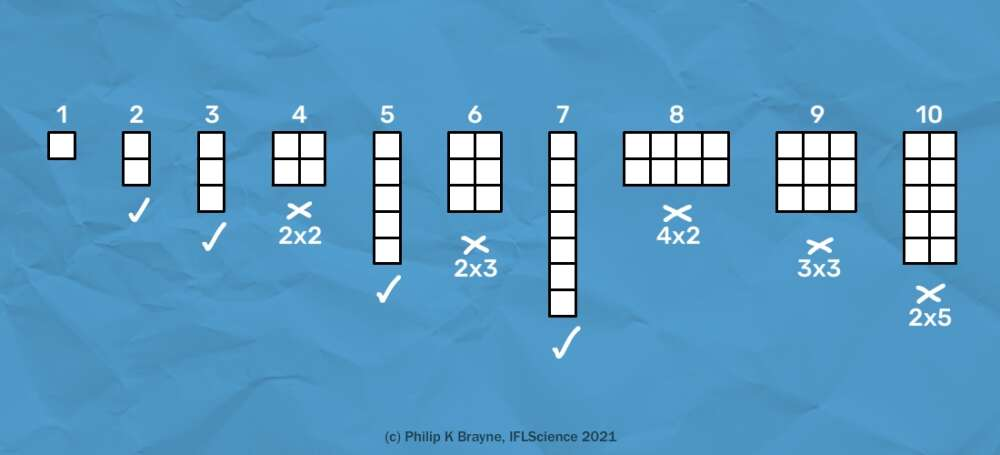Prime numbers can only be visualized as a column one block wide, but numbers which aren't prime can be visualized as squares or rectangles. IFLS

Now consider how many there are between zero and 100: 25.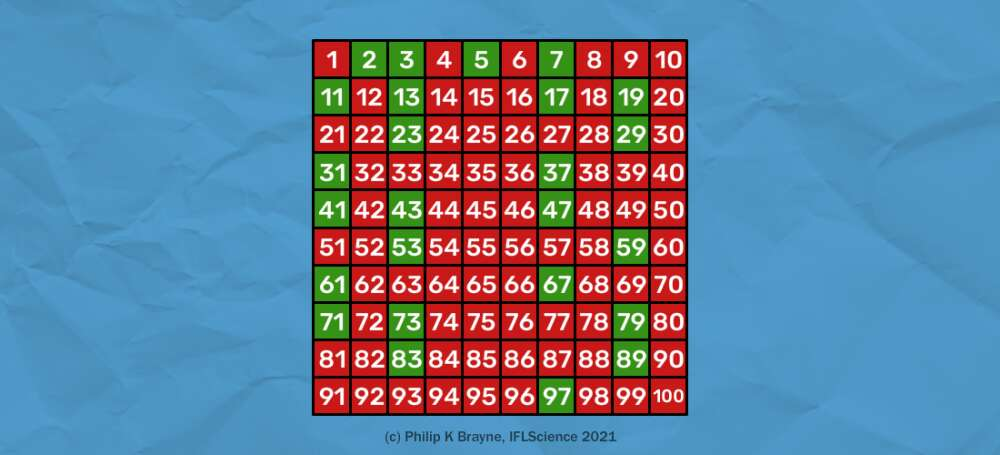Green numbers are prime; anything in red can be split into at least two factors. IFLS

Between zero and 1,000, you’ll find 168 prime numbers, and between zero and 10,000 (don’t worry, I won’t make you check) there are 1,229.

So each time we increase the size of our interval by a factor of ten, the amount of it that is given over to prime numbers goes from 40 percent to 25 percent, to 16.8 percent, to 12.29 percent. In other words: primes are getting “rarer”. And by 1793, when he was all of 16 years old, Gauss had figured out how.

“I soon recognized,” he wrote in a letter to his friend Johann Encke, “that behind all of its fluctuations, this frequency is on the average inversely proportional to the logarithm, so that the number of primes below a given bound n is approximately equal to ?dn/log(n).”

That rather off-hand remark, rewritten in modern mathematics, is now known as the Prime Number Theorem.

So much for the “average” behavior, but what about those “fluctuations” Gauss mentioned? Well, those are related to something called the zeta function – and this is where Riemann comes in.

Bernhard Riemann was a student of Gauss, and he made many important contributions to the world of math. His work impacted everything from calculus to differential geometry and even laid the groundwork for the development of general relativity, which is not bad for a guy who didn’t attend formal schooling until he was 14. In his short but impressive life, he only ever wrote one paper on number theory, but boy was it a doozy: in 1859, as a condition of his being elected to the Berlin Academy of Sciences, Riemann submitted a now-famous paper titled “On the number of primes less than a given magnitude”.

The zeta function, so-called because it is denoted by the Greek letter zeta, had originally been considered by Euler nearly a century beforehand.

What Riemann did with the zeta function, however, was completely different.

See it? That R has become a C. I know it doesn’t look like much, but that little change takes the zeta function from the real numbers to the complex numbers, and that is a very different function altogether. So important was this change that the function is now known as the Riemann zeta function, and many people aren’t aware Euler had anything to do with it at all (don’t feel too bad for old Euler though – he has enough stuff named after him already.)

Wait – complex numbers? What are they?

Ah yes – sorry. Complex numbers aren’t too difficult to wrap your head around, but there’s a decent chance you’ve not seen them before unless you did a math degree. Basically, there’s two types of numbers: real, and complex (well ok, there’s quaternions as well, but they’re not important right now so let’s not confuse things.)

A real number is pretty much any number you might think of if somebody says “think of a number”. Yes, even when you’re feeling cheeky and come up with something like ? or log(2). Basically, if you can see it anywhere on the number line, it’s a real number.

Then there are complex numbers. A good way to think of complex numbers is like a pair of co-ordinates on a graph. Along the bottom, we have the real number line. Up the side, we have what’s known as the imaginary number line, which is pretty much the same as the real number line except we write an “i” after each number.

This i is the imaginary unit, and its defining feature is that if you square it, you get negative one. That’s why complex numbers are different from reals: when you square a real number, you can only get positive answers. When you square complex numbers, you can get positive or negative answers.

There are a bunch of reasons to study complex numbers, but the one that’s important for us at the moment is what happens when you pop them into the Riemann zeta function.

Which is what?

So, whenever we have a function, a good question mathematicians like to ask is: where are the zeroes? Or in other words: what values can I put into this function to get an answer of zero?

Riemann calculated some of these zeroes in his 1859 paper, and he found that all of them had a real part equal to 1/2 – or, if you want to think of it in terms of our graph coordinates, they all lay on the same vertical line.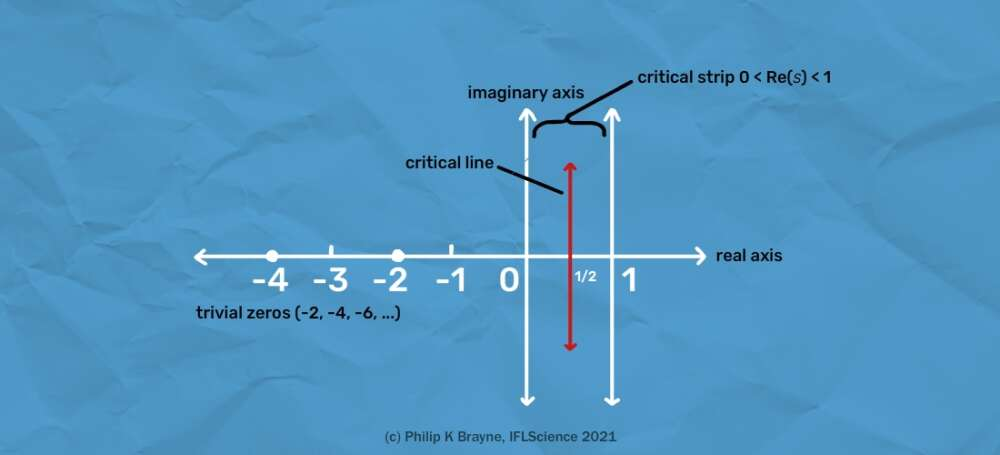Riemann Zeta Graph

In fact, Riemann thought it was likely that all of the zeta function’s infinite number of zeroes lay on this line.

And that’s the Riemann hypothesis?

That’s it! The Riemann hypothesis states that “The real part of every nontrivial zero of the Riemann zeta function is 1/2”.

It’s actually been shown that the first ten trillion zeroes do lie on this “critical line”, which is one reason why so many people think it must be true. But in math, experiments – even ten trillion of them – aren’t proof, and until the hypothesis is proven mathematically there’ll always be that chance that the ten trillion and one-th zero turns up somewhere different.

Strangely, Riemann didn’t seem to understand the groundbreaking implications of his hypothesis. He mentioned it casually as an unimportant aside, and moved on.

Why is it so important?

The Riemann hypothesis has been shown to be relevant in just about every area of math, and equivalent to an incredible range of seemingly unrelated conjectures. It has even turned up in crystals.

Hundreds of theorems depend on it being true, so there’s a lot riding on it. And of course, there’s the small matter of mathematicians themselves, who would probably have a collective identity crisis were the Riemann hypothesis shown to be false. As the mathematician Peter Sarnak said:

“If [the Riemann Hypothesis is] not true, then the world is a very different place. The whole structure of integers and prime numbers would be very different to what we could imagine. In a way, it would be more interesting if it were false, but it would be a disaster because we've built so much round assuming its truth.”

I heard somebody proved the Riemann hypothesis – is that true?

Well… probably not, no. After all, it’s been over 160 years, and not one of the very best mathematicians in the world has been able to crack it.

Every so often, somebody makes the headlines with a supposed “proof”, but so far none have been confirmed. In 2015, rumors started circulating that Nigerian mathematics professor Opeyemi Enoch had solved it, but they were almost immediately debunked.

In 2018 the renowned mathematician and physicist Sir Michael Atiyah announced he had a solution – but it didn’t hold up.

Most recently, Hyderabad physicist Kumar Eswaran was reported to have proven the hypothesis, but those reports were swiftly retracted when the Clay Institute announced the proof was invalid, and the million-dollar prize was still up for grabs.

Did you say a million dollars?

Yep – remember those “Millennium Prize” problems I mentioned earlier? The solution of any of them would win the responsible mathematician \$1,000,000. So far only one has been cracked – and it wasn’t the Riemann hypothesis.

Of course, any self-respecting mathematician would only be in it for the math, right?

Right! But on an unrelated note, what would be the best way to solve the Riemann hypothesis?

It depends who you ask! The truth is, we really don’t know – but given how many people have tried and failed already, it will probably come from somewhere unexpected, maybe even a brand new area of math altogether.

Of course, that’s assuming it can be solved at all. Mathematician Gregory Chaitin has suggested that a proof might not exist – ironically though, this would itself be impossible to prove!

So what’s the point in studying it then?

Look, it’s true that you’re unlikely to win a million dollars or solve a problem that nobody has been able to in over 160 years. But it’s not impossible. But really, the benefit of all these mathematicians working to find a proof that may not exist is what they find in the meantime.

It took 350 years to prove Fermat’s Last Theorem, but those 350 years were filled with mathematical innovations found by people chasing a solution. It’s only been 160 years for the Riemann hypothesis – who knows what math we have yet to discover?

## This Week in IFLScience

### ARTICLE POSTED IN

•• math,

• mathematics,

• numbers,

• Riemann Hypothesis,

• Riemann,

• complex numbers